# Clamper (electronics)

﻿
Clamper (electronics)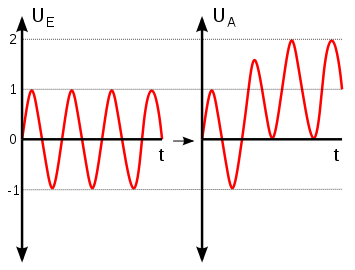Positive unbiased voltage clamping shifts the amplitude of the input waveform so that all parts of it are greater than 0 V

A clamper is an electronic circuit that prevents a signal from exceeding a certain defined magnitude by shifting its DC value. The clamper does not restrict the peak-to-peak excursion of the signal, but moves it up or down by a fixed value. A diode clamp (a simple, common type) relies on a diode, which conducts electric current in only one direction; resistors and capacitors in the circuit are used to maintain an altered dc level at the clamper output.

## General function

A clamping circuit (also known as a clamper) will bind the upper or lower extreme of a waveform to a fixed DC voltage level. These circuits are also known as DC voltage restorers. Clampers can be constructed in both positive and negative polarities. When unbiased, clamping circuits will fix the voltage lower limit (or upper limit, in the case of negative clampers) to 0 Volts. These circuits clamp a peak of a waveform to a specific DC level compared with a capacitively coupled signal which swings about its average DC level

## Types

Clamp circuits are categorised by their operation; negative or positive and biased and unbiased. A positive clamp circuit outputs a purely positive waveform from an input signal; it offsets the input signal so that all of the waveform is greater than 0 V. A negative clamp is the opposite of this - this clamp outputs a purely negative waveform from an input signal.

A bias voltage between the diode and ground offsets the output voltage by that amount.

For example, an input signal of peak value 5 V (VIN = 5 V) is applied to a positive clamp with a bias of 3 V (VBIAS = 3 V), the peak output voltage will be

VOUT = 2VIN + VBIAS
VOUT = 2 * 5 V + 3 V
VOUT = 13 V

### Positive unbiased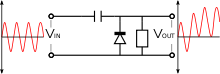A positive unbiased clamp

In the negative cycle of the input AC signal, the diode is forward biased and conducts, charging the capacitor to the peak positive value of VIN. During the positive cycle, the diode is reverse biased and thus does not conduct. The output voltage is therefore equal to the voltage stored in the capacitor plus the input voltage gain, so VOUT = 2VIN

### Negative unbiased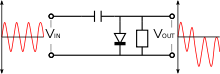A negative unbiased clamp

A negative unbiased clamp is the opposite of the equivalent positive clamp. In the positive cycle of the input AC signal, the diode is forward biased and conducts, charging the capacitor to the peak value of VIN. During the negative cycle, the diode is reverse biased and thus does not conduct. The output voltage is therefore equal to the voltage stored in the capacitor plus the input voltage again, so VOUT = -2VIN

### Positive biased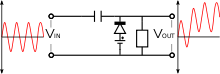A positive biased clamp

A positive biased voltage clamp is identical to an equivalent unbiased clamp but with the output voltage offset by the bias amount VBIAS. Thus, VOUT = 2VIN + VBIAS

### Negative biased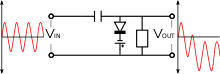A negative biased clamp

A negative biased voltage clamp is likewise identical to an equivalent unbiased clamp but with the output voltage offset in the negative direction by the bias amount VBIAS. Thus, VOUT = -2VIN - VBIAS

### Op-amp circuit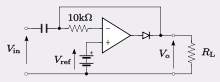Precision op-amp clamp circuit

The figure shows an op-amp clamp circuit with a non-zero reference clamping voltage. The advantage here is that the clamping level is at precisely the reference voltage. There is no need to take into account the forward volt drop of the diode (which is necessary in the preceding simple circuits as this adds to the reference voltage). The effect of the diode volt drop on the circuit output will be divided down by the gain of the amplifier, resulting in an insignificant error.

## Clamping for input protection

Clamping can be used to adapt an input signal to a device that cannot make use of or may be damaged by the signal range of the original input.

## Principles of operation

The schematic of a clamper reveals that it is a relatively simple device. The two components creating the clamping effect are a capacitor, followed by a diode in parallel with the load. The clamper circuit relies on a change in the capacitor’s time constant; this is the result of the diode changing current path with the changing input voltage. The magnitude of R and C are chosen so that$\tau = RC$ is large enough to ensure that the voltage across the capacitor does not discharge significantly during the diode's "Non conducting" interval. During the first negative phase of the AC input voltage, the capacitor in the positive clamper charges rapidly. As Vin becomes positive, the capacitor serves as a voltage doubler; since it has stored the equivalent of Vin during the negative cycle, it provides nearly that voltage during the positive cycle; this essentially doubles the voltage seen by the load. As Vin becomes negative, the capacitor acts as a battery of the same voltage of Vin. The voltage source and the capacitor counteract each other, resulting in a net voltage of zero as seen by the load.

## Biased versus non-biased

By using a voltage source and resistor, the clamper can be biased to bind the output voltage to a different value. The voltage supplied to the potentiometer will be equal to the offset from zero (assuming an ideal diode) in the case of either a positive or negative clamper (the clamper type will determine the direction of the offset. If a negative voltage is supplied to either positive or negative, the waveform will cross the x-axis and be bound to a value of this magnitude on the opposite side. Zener diodes can also be used in place of a voltage source and potentiometer, hence setting the offset at the Zener voltage.

## Examples

One common such clamping circuit is the DC restorer circuit in analog television receiver, which returns the voltage of the signal during the back porch of the line blanking period to 0 V. Since the back porch is required to be at 0 V on transmission, any DC or low frequency hum that has been induced onto the signal can be effectively removed via this method.

Wikimedia Foundation. 2010.

### Look at other dictionaries:

• Clipper (electronics) — Voltage clipping limits the voltage to a device without affecting the rest of the waveform In electronics, a clipper is a device designed to prevent the output of a circuit from exceeding a predetermined voltage level without distorting the… …   Wikipedia

• Electronic amplifier — A practical amplifier circuit An electronic amplifier is a device for increasing the power of a signal. It does this by taking energy from a power supply and controlling the output to match the input signal shape but with a larger amplitude. In… …   Wikipedia

• Oscar/Technische Verdienste — Der Oscar für technische Verdienste (Academy Technical Achievement Award) ist eine seit 1931 vergebenene Auszeichnung der Academy of Motion Picture Arts and Sciences und würdigt herausragende Leistungen auf dem Gebiet der Verbesserung technischer …   Deutsch Wikipedia

• C.O.P.S. — For other uses, see Cop (disambiguation). CyberCOPS redirects here. For the Japanese TV series, see Dennou Keisatsu Cybercop. C.O.P.S. Logo shown for C.O.P.S. Format Animated series …   Wikipedia